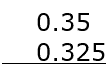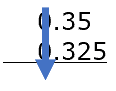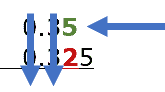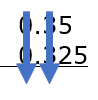# Comparing Decimals Worksheets

## All About These 15 Worksheets

These Comparing Decimals worksheets are designed to help students master the concept of comparing decimals. The worksheets include a variety of creative and engaging exercises to help students build their skills and confidence in comparing decimal numbers.

Each worksheet in the series includes a mix of problems with varying degrees of difficulty, designed to challenge and engage students at different skill levels. The problems require students to compare decimal numbers using comparison symbols such as greater than (>), less than(<), and equal to (=).

This series of worksheets is most suitable for students in grades 4 to 6. It can be used in the classroom as a supplement to the math curriculum or at home as a way for parents to help their children practice and reinforce their math skills.

Overall, these Comparing Decimals worksheets provide a comprehensive and engaging way for students to develop their skills and confidence in comparing decimal numbers. With these worksheets, students can build a solid foundation in math and develop the skills they need to succeed in higher-level math courses.

## How to Compare Decimal Values

Comparing decimal values is an essential skill in mathematics and everyday life. To compare decimal values, teachers can instruct their students to follow these steps:

1. Line Up the Decimal Points – When comparing two or more decimals, it’s helpful to write them vertically with their decimal points aligned. This makes it easier to compare the digits in each place value.
2. Compare the Whole Number Part – Start by comparing the whole number part (the digits to the left of the decimal point) of each number. If one whole number part is larger than the other, then that decimal is greater.
3. Compare Digits to the Right of the Decimal Point – If the whole number parts are equal, then compare the digits to the right of the decimal point, starting with the tenths place (the first digit to the right of the decimal point). If one decimal has a larger digit in the tenths place, then that decimal is greater.
4. Continue Comparing Digits – If the tenths place digits are equal, move on to the hundredths place (the second digit to the right of the decimal point), and so on. Continue this process until you find a digit that is different in the two decimals.
5. Adding Zeros, if necessary – If one decimal has more digits after the decimal point than the other, you can add zeros to the shorter decimal to make it easier to compare. For example, when comparing 0.5 and 0.50, add a zero to 0.5 to get 0.50. Since the two decimals are equal, adding zeros does not change their value.

Determine which decimal is greater or smaller. Once you find a digit that is different in the two decimals, the decimal with the larger digit in that place value is greater. If no different digits are found after comparing all the digits to the right of the decimal point, then the decimals are equal.

Let’s compare 0.35 and 0.325 using the steps above.

Line up the decimal points:Compare the whole number part: Both have 0 as the whole number part.Compare digits to the right of the decimal point: The tenths place has 3 for both numbers.Continue comparing digits: In the hundredths place, the first number has 5 while the second number has 2.Adding zeros, if necessary: No need to add zeros in this case.

Determine which decimal is greater or smaller: Since 5 is greater than 2 in the hundredths place, 0.35 is greater than 0.325.

## When Do We Compare Decimals In Our Daily Lives?

Comparing decimals is an important skill that we use in various aspects of our daily lives. Here are some common situations where we may need to compare decimals.

• Money: When dealing with currency, we often compare decimals to determine the cost of items, find the best deals, or calculate savings. For example, comparing the price per unit of products at a grocery store, or comparing interest rates on loans and credit cards.
• Measurements: Decimals are often used in measurements for length, weight, volume, or temperature. We may compare decimals when comparing the dimensions of objects, calculating the distance between two locations, or determining the difference in weight or temperature.
• Time: Although time is typically expressed in hours, minutes, and seconds, it can also be represented in decimal form. For example, we may need to compare the time taken to complete tasks, the average speed of vehicles, or the durations of events.
• Sports and fitness: In sports, decimals are used to express statistics, scores, or timings. We may compare decimals when determining the winner of a race, tracking personal bests, or analyzing the performance of athletes or teams.
• Data analysis: Decimals are frequently used in data analysis to represent percentages, rates, or ratios. We may need to compare decimals when analyzing survey results, evaluating financial data, or making informed decisions based on data trends.
• Cooking and baking: Recipes often include measurements in decimal form, especially when using the metric system. We may compare decimals when converting between measurement units or adjusting recipe quantities.
• Grades and test scores: In education, decimals are used to represent grades or test scores. We may compare decimals to determine a student’s progress, evaluate class performance, or rank students based on their scores.
• Electronics and technology: Decimals are used to represent various aspects of electronic devices and technology, such as processor speeds, storage capacity, or screen resolution. We may compare decimals when choosing between different devices or evaluating their performance.

In summary, comparing decimals is an essential skill that allows us to make informed decisions, evaluate data, and solve problems in a variety of contexts.